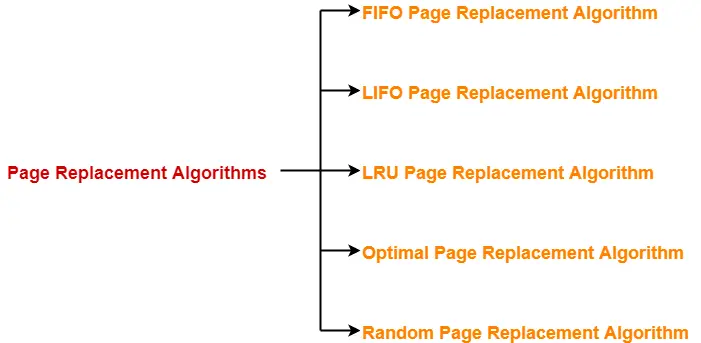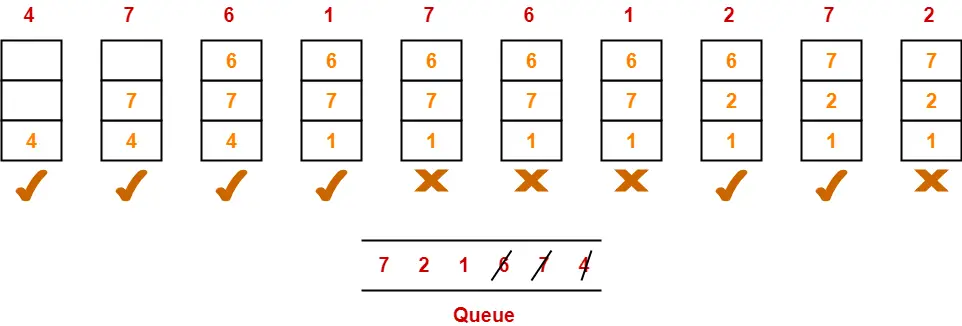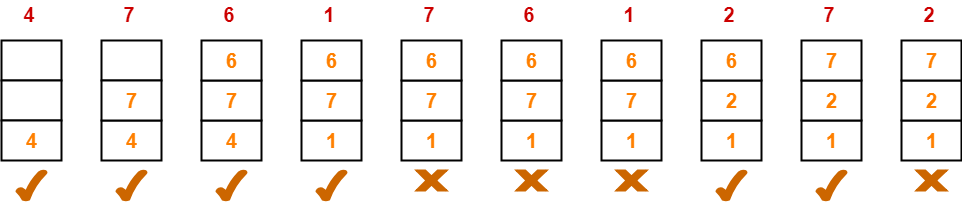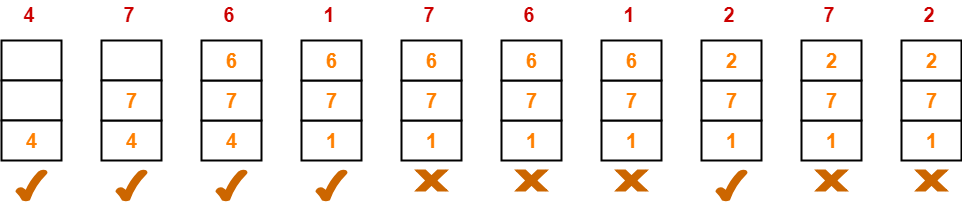# Page Replacement Algorithms | Page Fault

## Page Fault in OS-

Before you go through this article, make sure that you have gone through the previous article on Page Fault in OS.

We have discussed-

• A page fault occurs when a page referenced by the CPU is not found in the main memory.
• The required page has to be brought from the secondary memory into the main memory.
• A page has to be replaced if all the frames of main memory are already occupied.

## Page Replacement-

 Page replacement is a process of swapping out an existing page from the frame of a main memory and replacing it with the required page.

Page replacement is required when-

• All the frames of main memory are already occupied.
• Thus, a page has to be replaced to create a room for the required page.

## Page Replacement Algorithms-

Page replacement algorithms help to decide which page must be swapped out from the main memory to create a room for the incoming page.

Various page replacement algorithms are-1. FIFO Page Replacement Algorithm
2. LIFO Page Replacement Algorithm
3. LRU Page Replacement Algorithm
4. Optimal Page Replacement Algorithm
5. Random Page Replacement Algorithm

 A good page replacement algorithm is one that minimizes the number of page faults.

## FIFO Page Replacement Algorithm-

• As the name suggests, this algorithm works on the principle of “First in First out“.
• It replaces the oldest page that has been present in the main memory for the longest time.
• It is implemented by keeping track of all the pages in a queue.

## LIFO Page Replacement Algorithm-

• As the name suggests, this algorithm works on the principle of “Last in First out“.
• It replaces the newest page that arrived at last in the main memory.
• It is implemented by keeping track of all the pages in a stack.

### NOTE

Only frame is used for page replacement during entire procedure after all the frames get occupied.

## LRU Page Replacement Algorithm-

• As the name suggests, this algorithm works on the principle of “Least Recently Used“.
• It replaces the page that has not been referred by the CPU for the longest time.

## Optimal Page Replacement Algorithm-

• This algorithm replaces the page that will not be referred by the CPU in future for the longest time.
• It is practically impossible to implement this algorithm.
• This is because the pages that will not be used in future for the longest time can not be predicted.
• However, it is the best known algorithm and gives the least number of page faults.
• Hence, it is used as a performance measure criterion for other algorithms.

## Random Page Replacement Algorithm-

• As the name suggests, this algorithm randomly replaces any page.
• So, this algorithm may behave like any other algorithm like FIFO, LIFO, LRU, Optimal etc.

## Problem-01:

A system uses 3 page frames for storing process pages in main memory. It uses the First in First out (FIFO) page replacement policy. Assume that all the page frames are initially empty. What is the total number of page faults  that will occur while processing the page reference string given below-

4 , 7, 6, 1, 7, 6, 1, 2, 7, 2

Also calculate the hit ratio and miss ratio.

## Solution-

Total number of references = 10From here,

Total number of page faults occurred = 6

### Calculating Hit ratio-

Total number of page hits

= Total number of references – Total number of page misses or page faults

= 10 – 6

= 4

Thus, Hit ratio

= Total number of page hits / Total number of references

= 4 / 10

= 0.4 or 40%

### Calculating Miss ratio-

Total number of page misses or page faults = 6

Thus, Miss ratio

= Total number of page misses / Total number of references

= 6 / 10

= 0.6 or 60%

Alternatively,

Miss ratio

= 1 – Hit ratio

= 1 – 0.4

= 0.6 or 60%

## Problem-02:

A system uses 3 page frames for storing process pages in main memory. It uses the Least Recently Used (LRU) page replacement policy. Assume that all the page frames are initially empty. What is the total number of page faults  that will occur while processing the page reference string given below-

4 , 7, 6, 1, 7, 6, 1, 2, 7, 2

Also calculate the hit ratio and miss ratio.

## Solution-

Total number of references = 10From here,

Total number of page faults occurred = 6

In the similar manner as above-

• Hit ratio = 0.4 or 40%
• Miss ratio = 0.6 or 60%

## Problem-03:

A system uses 3 page frames for storing process pages in main memory. It uses the Optimal page replacement policy. Assume that all the page frames are initially empty. What is the total number of page faults  that will occur while processing the page reference string given below-

4 , 7, 6, 1, 7, 6, 1, 2, 7, 2

Also calculate the hit ratio and miss ratio.

## Solution-

Total number of references = 10From here,

Total number of page faults occurred = 5

In the similar manner as above-

• Hit ratio = 0.5 or 50%
• Miss ratio = 0.5 or 50%

To gain better understanding about Page Replacement Algorithms,

Watch this Video Lecture

Next Article- Practice Problems On Page Fault

Get more notes and other study material of Operating System.

Watch video lectures by visiting our YouTube channel LearnVidFun.

SummaryArticle Name
Page Replacement Algorithms | Page Fault
Description
When page fault occurs, page replacement algorithms help to decide which page must be replaced. FIFO Page Replacement Algorithm, LRU Page Replacement Algorithm, Optimal Page Replacement Algorithm are famous Page Replacement Algorithms.
Author
Publisher Name
Gate Vidyalay
Publisher Logo